edHelper.com Math Worksheets Time Worksheets 1st Grade Telling TimeWorksheets, Lessons, and Printables

Telling Time Math Center (easier - hour hand does not move between numbers)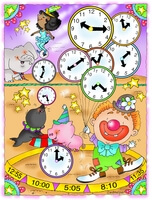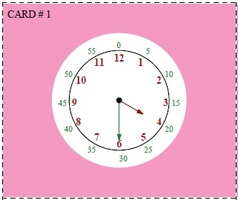Minutes shown on clock (Grades K-1) Normal clock - just hours shown on clock (Grades 1-3)

Telling Time Math Center (more difficult - hour hand moves with minutes like a real clock)Minutes shown on clock (Grades K-1) Normal clock - just hours shown on clock (Grades 1-3)

Telling Time Activity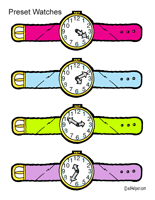Which Watch

Writing Numbers on a Clock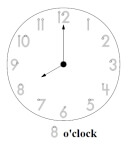Trace all the numbers on a clock

Book Units
Benjamin Banneker: Pioneering Scientist (Grades 2-5)

Missing Number on a Clock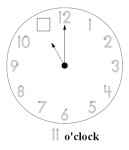Trace numbers on clock and fill in the missing number

Easy Clock and Time Matching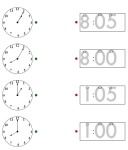Clock Matching: Hours Clock Matching: On the hour and five minutes past given (same hour used)

Clock Activity Center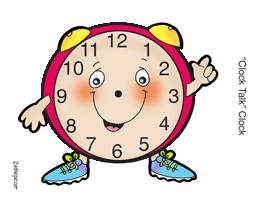Clock Talk

Hour
Write the time (____ o'clock; half past ____)
Write the time (____:____)
Write the time two ways
Show the time on the clock
Write the time two ways (smaller clocks)

Half-Hour
Write the time (half past ____)
Write the time (____:____)
Write the time two ways
Show the time on the clock
Write the time two ways (smaller clocks)

Mixed: Hour and Half-Hour (larger clocks)
Write the time (half past ____ and ____ o'clock)
Write the time (____:____)
Write the time two ways
Show the time on the clock

Mixed: Hour and Half-Hour (smaller clocks)
Write the time (half past ____ and ____ o'clock)
Write the time (____:____)
Write the time two ways
Show the time on the clock
Circle the correct time (2 choices)

Review
Telling Time Mixed Review

Leveled Books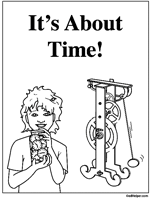It's About Time!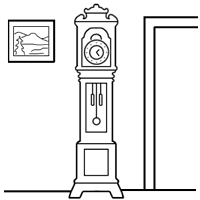My Grandmother's Grandfather Clock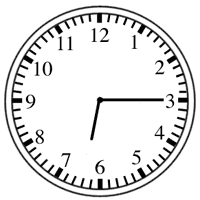What Time Is It?

Understanding Time Words
Time Words (Half-Hour and Hour)
Time Words (Five Minutes)
Time Words (Any Time)
Time Words (Five Minutes; May Include "Quarter To")
Time Words (Any Time; May Include "Quarter To")

Telling Time

Have a suggestion or would like to leave feedback?